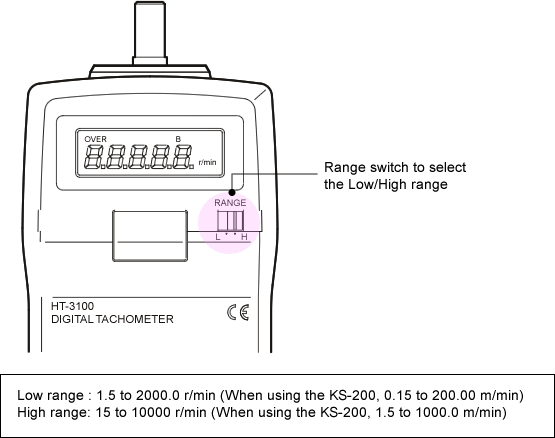Tachometer FAQWhat is the measurement range of the HT-3100?The HT-3100 Hand Tachometer has a range switch to select the low or high measurement range.Incidentally, when using the KS-200 Circumference ring, it is necessary to multiply the displayed reading by 0.1 to get the actual measurement.

Ex.)
If the displayed reading is "123", the actual measurement would be "12.3 m/min".
If the displayed reading is "523", the actual measurement would be "52.3 m/min".
If the displayed reading is "12.3", the actual measurement would be "1.23 m/min".

Revised:2007/02/19